# Texas Go Math Grade 4 Lesson 1.4 Answer Key Round Numbers

Refer to our Texas Go Math Grade 4 Answer Key Pdf to score good marks in the exams. Test yourself by practicing the problems from Texas Go Math Grade 4 Lesson 1.4 Answer Key Round Numbers.

## Texas Go Math Grade 4 Lesson 1.4 Answer Key Round Numbers

Essential Question

How can you round numbers?
The general rules for the rounding of numbers are:
a. If the number you are rounding is followed by 5, 6, 7, 8, or 9, round the number up.
Example:
38 rounded to the nearest ten is 40.
b. If the number you are rounding is followed by 0, 1, 2, 3, or 4, round the number down.
Example:
33 rounded to the nearest ten is 30.

In May 2008, the Mount Rushmore National Monument in South Dakota welcomed 138,202 visitors. A website reported that about 1 hundred thousand people visited the park during that month. Was the estimate reasonable?

• Underline what you are asked to find.
• Circle the information you will use.

An estimate tells you about how many or about how much. It is close to an exact amount. You can round a number to find an estimate.

One Way Use a number

To round a number to the nearest hundred thousand, find the hundred thousand it is between.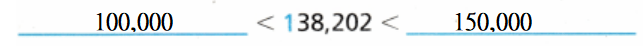Use a number line to see which hundred thousand 138,202 is closest to138,202 is closer to 150,000 than 100,000
Hence,
1 hundred thousand is not a reasonable estimate for 138,202.

Math Talk

Mathematical Process:
Is 155,000 closer to 100,000 or 200,000? Explain
The given number is: 155,000
Now,
We know that,
If the number you are rounding is followed by 5, 6, 7, 8, or 9, round the number up.
So,
155,000 should be close to 200,000 since 155 is close to 200 than 100
Hence, from the above,
We can conclude that
155,000 is closer to 200,000

Question 1.
What number is halfway between 100,000 and 200,000?
The given numbers are: 100,000 and 200,000
Now,
We know that,
The half of the 2 numbers = $$\frac{a + b}{2}$$
Where,
a and b are 2 numbers
So,
The half of 100,000 and 200,000 = $$\frac{100,000 + 200,000}{2}$$
= $$\frac{300,000}{2}$$
= 150,000
Hence, from the above,
We can conclude that
150,000 is halfway between 100,000 and 200,000

Question 2.
How does knowing where the halfway point helps you find which hundred thousand 138,202 is closest to? Explain.
The given number line is:Now,
From the above number line,
We can observe that
138,202 is closer to 150,000
Now,
We know that
150,000 is halfway of 100,000 and 200,000
Hence, from the above,
We can conclude that
138,202 is closer to the halfway point by using the number line

Another Way Use place value.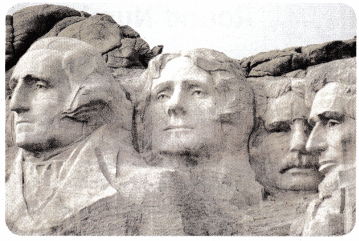Mount Rushmore is located 5,725 feet above sea level. About how high is Mount Rushmore above sea level, to the nearest thousand feet?

To round a number to the nearest thousand, find the thousands it is between.Look at the digit in the place-value position to the right.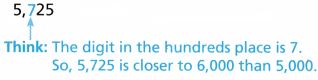Hence, from the above,
We can conclude that
Mount Rushmore is about 6,000 feet above sea level.

Math Talk

Mathematical Process
Explain how you know 5,700 is closer to 6,000 than to 5,000.
We know that,
The half of the given numbers = $$\frac{a + b}{2}$$
So,
The halfway of 5,000 and 6,000 = $$\frac{5,000 + 6,000}{2}$$
= $$\frac{11,000}{2}$$
= 5,500
So,
5,700 > 5,500
So,
5,700 is closer to 6,000
Hence, from the above,
We can conclude that
5,700 is closer to 6,000 than 5,000 since the halfway of 5,000 and 6,000 is less than 5,700

Question 3.
What is 250,000 rounded to the nearest hundred thousand? Explain.
The given number is: 250,000
Now,
We know that,
If the number is greater than or equal to the halfway of the neasrest hundred thousand of the given number, then the given number is rounded to the latter number
Now,
The numbers that are closer to hundred thousand for 250,000 are: 200,000 and 300,000
So,
The halfway of 200,000 and 300,000 = $$\frac{200,000 + 300,000}{2}$$
= $$\frac{500,000}{2}$$
= 250,000
Hence, from the above,
We can conclude that
300,000 is the number that 250,000 is rounded to the nearest hundred thousand

Share and Show

Question 1.
Suppose 255,113 people live in a city. Rounded to the nearest hundred thousand, about how many people live in the city? Explain.
_____________ > 255,113 > _____________
The given number is: 255,113
Now,
We know that,
If the number is greater than or equal to the halfway of the neasrest hundred thousand of the given number, then the given number is rounded to the latter number
Now,
The numbers that are closer to hundred thousand for 255,113 are: 200,000 and 300,000
So,
The halfway of 200,000 and 300,000 = $$\frac{200,000 + 300,000}{2}$$
= $$\frac{500,000}{2}$$
= 250,000
Hence, from the above,
We can conclude that
The number of people live in the city is about 300,000 people

Round to the place value of the underlined digit.

Question 2.
934,567
The given number is: 934,567
Now,
We know that,
If the number you are rounding is followed by 5, 6, 7, 8, or 9, round the number up.
If the number you are rounding is followed by 0, 1, 2, 3, or 4, round the number down.
Hence, from the above,
We can conclude that
934,567 is rounded to 930,000

Question 3.
641,267
The given number is: 641,267
Now,
We know that,
If the number you are rounding is followed by 5, 6, 7, 8, or 9, round the number up.
If the number you are rounding is followed by 0, 1, 2, 3, or 4, round the number down.
Hence, from the above,
We can conclude that
641,267 is rounded to 640,000

Question 4.
1,234,890
The given number is: 1,234,890
Now,
We know that,
If the number you are rounding is followed by 5, 6, 7, 8, or 9, round the number up.
If the number you are rounding is followed by 0, 1, 2, 3, or 4, round the number down.
Hence, from the above,
We can conclude that
1,234,890 is rounded to 1,230,000

Question 5.
347,456
The given number is: 347,456
Now,
We know that,
If the number you are rounding is followed by 5, 6, 7, 8, or 9, round the number up.
If the number you are rounding is followed by 0, 1, 2, 3, or 4, round the number down.
Hence, from the above,
We can conclude that
347,456 is rounded to 350,000

Problem Solving

Question 6.
H.O.T.
Multi-Step Apply The number 2,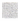00 is missing a digit. The number rounded to the nearest thousand is 3,000. List all of the possibilities for the missing digit. Explain your answer.
It is given that
The number 2,00 is missing a digit. The number rounded to the nearest thousand is 3,000
Now,
We know that,
If the number you are rounding is followed by 5, 6, 7, 8, or 9, round the number up.
If the number you are rounding is followed by 0, 1, 2, 3, or 4, round the number down.
So,
The missing digit should be: 5,6,7,8, and 9 since the given number rounded to 3,000
Hence, from the above,
We can conclude that
The list of all the possibilities for the missing digit is: 5, 6, 7, 8, and 9

Question 7.
H.O.T.
Multi-Step Evaluate Reasonableness The 2008 population of Wyoming was counted as 532,668 people. What is a reasonable estimate of the 2008 population of Wyoming? Explain.
It is given that
The 2008 population of Wyoming was counted as 532,668 people
Now,
We know that,
If the number you are rounding is followed by 5, 6, 7, 8, or 9, round the number up.
If the number you are rounding is followed by 0, 1, 2, 3, or 4, round the number down.
So,
The reasonable estimate of 532,668 is: 530,000
Hence, from the above,
We can conclude that
The reasonable estimate of the 2008 population of Wyoming is: 530,000

Question 8.
What is the greatest whole number that rounds to the number 277,300? What is the least whole number?
The given whole number is: 277,300
Now,
We know that,
If the number you are rounding is followed by 5, 6, 7, 8, or 9, round the number up.
If the number you are rounding is followed by 0, 1, 2, 3, or 4, round the number down.
So,
The reasonable estimates of 277,300 is:
200,000 < 277,300 < 300,000
Hence, from the above,
We can conclude that
The greatest whole number that rounds to the number 277,300 is: 300,000
The least whole number that rounds to the number 277,300 is: 200,000

Question 9.
Sarah rounded the number 4,583,583 to 4,500,000 when her teacher asked her to round to the nearest hundred thousand. Jamal rounded the same number to 4,600,000 when asked to round to the nearest hundred thousand. Who is correct? Explain the error that was made.
It is given that
Sarah rounded the number 4,583,583 to 4,500,000 when her teacher asked her to round to the nearest hundred thousand. Jamal rounded the same number to 4,600,000 when asked to round to the nearest hundred thousand
Now,
We know that,
If the number you are rounding is followed by 5, 6, 7, 8, or 9, round the number up.
If the number you are rounding is followed by 0, 1, 2, 3, or 4, round the number down.
So,
4,583,583 is closer to 4,600,000 than 4,500,000
Hence, from the above,
We can conclude that
Jamal is correct
The error made is that 4,583,383 is greater than halfway of 4,000,000 and 5,000,000 and so, the number should be the latter number i.e., greater than 4,583,583

Question 10.
What number is halfway between 34,000 and 35,000?
The given numbersa re: 34,000 and 35,000
Now,
We know that,
The halfway of the given numbers = $$\frac{a + b}{2}$$
So,
The halfway of 34,000 and 35,000 = $$\frac{34,000 + 35,000}{2}$$
= $$\frac{69,000}{2}$$
= 34,500
Hence, from the above,
We can conclude that
34,500 is halfway between 34,000 and 35,000

Question 11.
The number of dogs at The Great North Dog Walk in 2011, rounded to the nearest thousand, is 23,000. Which could be the exact number of dogs?
(A) 22,742
(B) 23,500
(C) 23,603
(D) 22,455
It is given that
The number of dogs at The Great North Dog Walk in 2011, rounded to the nearest thousand, is 23,000
Now,
We know that,
If the number you are rounding is followed by 5, 6, 7, 8, or 9, round the number up.
If the number you are rounding is followed by 0, 1, 2, 3, or 4, round the number down.
So,
The exact number should be greater than or equal to 22,500
Hence, from the above,
We can conclude that
The exact number of dogs is: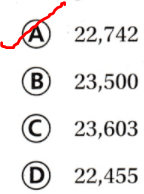Question 12.
A sports website had 2,684,735 visitors last year. Jerry rounded the number of visitors to the nearest hundred thousand for a newspaper article. Which number shows how he rounded 2,684,735?
(A) 2,684,400
(B) 2,680,000
(C) 2,690,000
(D) 2,700,000
It is given that
A sports website had 2,684,735 visitors last year. Jerry rounded the number of visitors to the nearest hundred thousand for a newspaper article
Now,
We know that,
If the number you are rounding is followed by 5, 6, 7, 8, or 9, round the number up.
If the number you are rounding is followed by 0, 1, 2, 3, or 4, round the number down.
So,
The number that is closer to 2,684,735 is: 2,684,000
Hence, from the above,
We can conclude that
The number that shows the rounded number of 2,684,735 is:Question 13.
Multi-Step Rachael rounded 16,473 to the nearest hundred. Then she rounded her answer to the nearest thousand. What is the final number?
(A) 16,500
(B) 17,000
(C) 20,000
(D) 16,000
It is given that
Rachael rounded 16,473 to the nearest hundred. Then she rounded her answer to the nearest thousand
Now,
We know that,
If the number you are rounding is followed by 5, 6, 7, 8, or 9, round the number up.
If the number you are rounding is followed by 0, 1, 2, 3, or 4, round the number down.
So,
The number that is to the nearest hundred for 16,473 is: 16,500
The number that is to the nearest thousand for 16,473 is: 17,000
Hence, from the above,
We can conclude that
The final number is:TEXAS Test prep

Question 14.
About 300,000 people attended a festival. Which number could be the exact number of people that attended the festival?
(A) 389,001
(B) 249,899
(C) 252,348
(D) 351,213
It is given that
About 300,000 people attended a festival
Now,
We know that,
If the number you are rounding is followed by 5, 6, 7, 8, or 9, round the number up.
If the number you are rounding is followed by 0, 1, 2, 3, or 4, round the number down.
So,
The exact number should be greater than or equal to 250,000
Hence, from the above,
We can conclude that
The exact number of people that attended the festival is:Texas Go Math Grade 4 Lesson 1.4 Homework and Practice Answer Key

Question 1.
The number 5,00 is missing a digit. The number rounded to the nearest thousand is 5,000. List all of the possibilities for the missing digit. Explain your answer.
It is given that
The number 5,00 is missing a digit. The number rounded to the nearest thousand is 5,000
Now,
We know that,
If the number you are rounding is followed by 5, 6, 7, 8, or 9, round the number up.
If the number you are rounding is followed by 0, 1, 2, 3, or 4, round the number down.
So,
The missing digit for 5,000 is: 0, 1, 2, 3, and 4
Hence, from the above,
We can conclude that
The list of all of the possibilities for the missing digit is: 0, 1, 2, 3, and 4

Question 2.
Andy and Elizabeth each rounded 2,639,456 to the nearest ten-thousand. Andy got 2,640,000. Elizabeth got 2,630,000. Who is correct? Explain the error that was made.
It is given that
Andy and Elizabeth each rounded 2,639,456 to the nearest ten-thousand. Andy got 2,640,000. Elizabeth got 2,630,000
Now,
The given number is: 2,639,456
Now,
We know that,
If the number you are rounding is followed by 5, 6, 7, 8, or 9, round the number up.
If the number you are rounding is followed by 0, 1, 2, 3, or 4, round the number down.
So,
2,639,456 is closest to 2,640,000 since 639 is greater than 635
Hence, from the above,
We can conclude that
Andy is correct
The error made is that since 2,639,456 is greater than the halfway between 2,600,000 and 2,700,000, the given number must not be close to 2,630,000

Question 3.
What is the greatest whole number that rounds to 67400? What is the least whole number?
The given number is: 67,400
Now,
We know that,
If the number you are rounding is followed by 5, 6, 7, 8, or 9, round the number up.
If the number you are rounding is followed by 0, 1, 2, 3, or 4, round the number down.
So,
The range of the numbers that 67,400 is in between:
67,000 < 67,400 < 68,000
Hence, from the above,
We can conclude that
The greatest whole number is: 68,000
The least whole number is: 67,000

Question 4.
What number is halfway between 170,000 and 180,000?
The given numbers are: 170,000 and 180,000
Now,
We know that,
The halfway of the given numbers = $$\frac{a + b}{2}$$
So,
The halfway of 170,000 and 180,000 = $$\frac{170,000 + 180,000}{2}$$
= $$\frac{350,000}{2}$$
= 175,000
Hence, from the above,
We can conclude that
175,000 is halfway between 170,000 and 180,000

Problem Solving

Question 5.
A shopping channel shipped 467,023 packages last year. What is a reasonable estimate of the number of packages shipped? Explain.
It is given that
A shopping channel shipped 467,023 packages last year
Now,
We know that,
If the number you are rounding is followed by 5, 6, 7, 8, or 9, round the number up.
If the number you are rounding is followed by 0, 1, 2, 3, or 4, round the number down.
So,
467,023 is closer to 470,000
Hence, from the above,
We can conclude that
The reasonable estimate of the number of packages shipped is: 470,000 packages

Question 6.
A parking lot covers 32,420 square feet. What is its area to the nearest thousand feet? Explain how you round the number.
It is given that
A parking lot covers 32,420 square feet
Now,
We know that,
If the number you are rounding is followed by 5, 6, 7, 8, or 9, round the number up.
If the number you are rounding is followed by 0, 1, 2, 3, or 4, round the number down.
So,
32,420 is closest to 32,000
Hence, from the above,
We can conclude that
The area of the parking lot to the nearest thousand feet is: 32,000 square feet

Lesson Check:

Question 7.
The population of Forestville is 83,277. What is the population rounded to the nearest thousand?
(A) 83,000
(B) 80,000
(C) 84,000
(D) 90,000
It is given that
The population of Forestville is 83,277
Now,
We know that,
If the number you are rounding is followed by 5, 6, 7, 8, or 9, round the number up.
If the number you are rounding is followed by 0, 1, 2, 3, or 4, round the number down.
So,
The number that is closest to 83,277 is: 83,000
Hence, from the above,
We can conclude that
The population of Forestville that is rounded to the nearest thousand is: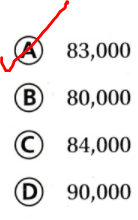Question 8.
To the nearest hundred thousand, there were 700,000 visitors to an amusement park last year. Which could be the exact number of visitors?
(A) 784,312
(B) 756,107
(C) 685,280
(D) 641,888
It is given that
To the nearest hundred thousand, there were 700,000 visitors to an amusement park last year
Now,
We know that,
If the number you are rounding is followed by 5, 6, 7, 8, or 9, round the number up.
If the number you are rounding is followed by 0, 1, 2, 3, or 4, round the number down.
So,
The number that should be closest to 700,000 should be greater than or equal to 650,000
Hence, from the above,
We can conclude that
The exact number of visitors is:Question 9.
What is the greatest whole number that rounds to 54,300?
(A) 53,399
(B) 54,349
(C) 53,999
(D) 54,299
The given number is: 54,300
Now,
We know that,
If the number you are rounding is followed by 5, 6, 7, 8, or 9, round the number up.
If the number you are rounding is followed by 0, 1, 2, 3, or 4, round the number down.
So,
The whole number that is closest to 54,300 should have the digits 0, 1, 2, 3, and 4
Hence, from the above,
We can conclude that
The greatest whole number that rounds to 54,300 is: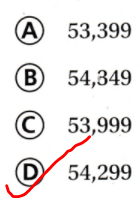Question 10.
The number 15,56 rounded to the nearest thousand is 15,000. Which numbers could be the missing digit?
(A) 1, 2, 3, 4, 5
(B) 1, 2, 3, 4, 5, 6
(C) 0, 1, 2, 3, 4, 5
(D) 0, 1, 2, 3, 4
It is given that
The number 15,56 rounded to the nearest thousand is 15,000
Now,
We know that,
If the number you are rounding is followed by 5, 6, 7, 8, or 9, round the number up.
If the number you are rounding is followed by 0, 1, 2, 3, or 4, round the number down.
So,
The digits for the given number should be: 0, 1, 2, 3, and 4
Hence, from the above,
We can conclude that
The numbers that could be the missing digit is:Question 11.
Multi-Step A male elephant weighs 6,708 pounds. A female elephant weighs 5,823 pounds. To the nearest hundred, what is the total weight of the two elephants?
(A) 7,000 pounds
(B) 12,500 pounds
(C) 6,000 pounds
(D) 13,000 pounds
It is given that
A male elephant weighs 6,708 pounds. A female elephant weighs 5,823 pounds
So,
The total weight of the elephants = 6,708 + 5,823
= 12,531 Pounds
Now,
We know that,
If the number you are rounding is followed by 5, 6, 7, 8, or 9, round the number up.
If the number you are rounding is followed by 0, 1, 2, 3, or 4, round the number down.
So,
12,531 is close to 12,500
Hence, from the above,
We can conclude that
The total weight of the elephants to the nearest hundred is: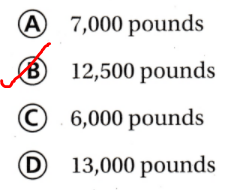Question 12.
Multi-Step To the nearest hundred, a factory-produced 3,600 jars of applesauce on Thursday and 4,200 jars of applesauce on Friday. To the nearest thousand, how many jars of applesauce did they produce on the two days?
(A) 7,800
(B) 7,000
(C) 8,400
(D) 8,000
It is given that
To the nearest hundred, a factory-produced 3,600 jars of applesauce on Thursday and 4,200 jars of applesauce on Friday
So,
The total number of jars of applesauce did they produce on two days = 3,600 + 4,200
= 7,800 jars
Now,
We know that,
If the number you are rounding is followed by 5, 6, 7, 8, or 9, round the number up.
If the number you are rounding is followed by 0, 1, 2, 3, or 4, round the number down.
So,
7,800 is close to 8,000
Hence, from the above,
We can conclude that
To the nearest thousand, the number of jars of applesauce did they produce on the two days is:Scroll to Top